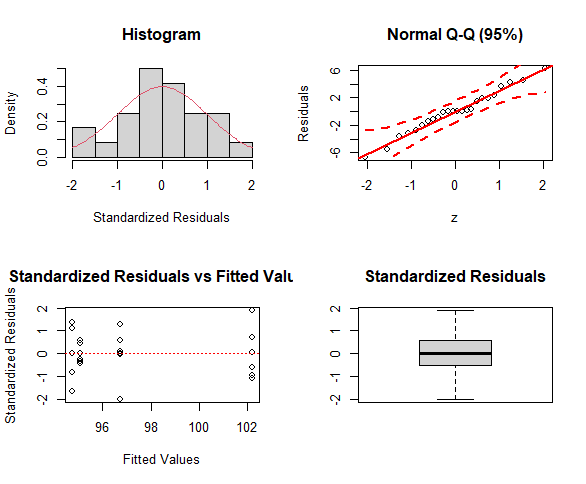## ExpDes: An R Package for ANOVA

Analysis of variance (ANOVA) is an usual way for analysing experiments. However, depending on the design and/or the analysis scheme, it can be a hard task. ExpDes, acronym for Experimental Designs, is a package that intends to turn such task easier. Devoted to fixed models and balanced experiments, ExpDes allows the user to deal with additional treatments in a single run, several experiment designs and exhibits standard and easy-to-interpret outputs.

``` `````` ```The main purpose of the package ExpDes is to analyze simple experiments under completely randomized designs (crd()), randomized block designs (cbd()) and Latin square designs (latsd()). Also enables the analysis of treatments in a factorial design with 2 and 3 factors (fat2.crd(), fat3.crd(), fat2.rbd(), fat3.rbd()) and also the analysis of split-plot designs (split2.crd(), split2.rbd()). Other functionality is analyzing experiments with one additional treatments on completely randomized design and randomized blocks design with 2 or 3 factors (fat2.ad.crd(), fat2.ad.rbd(), fat3.ad.crd() and fat3.ad.crd()).

``` `````` ```After loading the package and reading and attaching the data, a single command is required to analyze any situation. For instance, consider a completely randomized design for a qualitative factor. ``` ``` ``` ```
` crd(treat, resp, quali = TRUE, mcomp = "tukey") `
``` `````` ```For multiple comparison used Tukey´s test. Other tests where implements in package. For factor quantitative is possible used models regression linear and non linear. ``` `````` ``` ``` ```
` crd(trat, resp, quali = FALSE, sigF = 0.05) `
``` `````` `````` ```Output: ``` `````` ``` ``` ```
```---------------------------------------------------------------
Analysis of Variance Table
---------------------------------------------------------------
DF      SS      MS      Fc      Pr>Fc
Treatament  3   214.88  71.626  6.5212  0.0029622
Residuals   20  219.67  10.984
Total       23  434.55
---------------------------------------------------------------
CV = 3.41 %

---------------------------------------------------------------
Shapiro-Wilk normality test
p-value:  0.91697
According to Shapiro-Wilk normality test at 5% of significance,
residuals can be considered normal.
---------------------------------------------------------------

---------------------------------------------------------------
Homogeneity of variances test
p-value:  0.1863216
According to the test of bartlett at 5% of significance,
residuals can be considered homocedastic.
---------------------------------------------------------------

Tukey's test
---------------------------------------------------------------
Groups  Treatments  Means
a            A    102.1983
b           B    96.74333
b           C    95.05833
b           D    94.74333
---------------------------------------------------------------
```
``` `````` `````` ``` ``` ```
``` a <- crd(treat, resp)
plotres(a) ```
``` `````` `````` `````` ```The object “a” contains information about residuals, means, among others. In this way, graphs of averages and other analyzes can be performed. Grafical ExpDes (GExpDes) expansions are being worked on and new features are in progress. Information in 10.4236/am.2014.519280 and https://cran.r-project.org/web/packages/ExpDes/index.html.

``` ```